## Cap percentage values between 0 and 100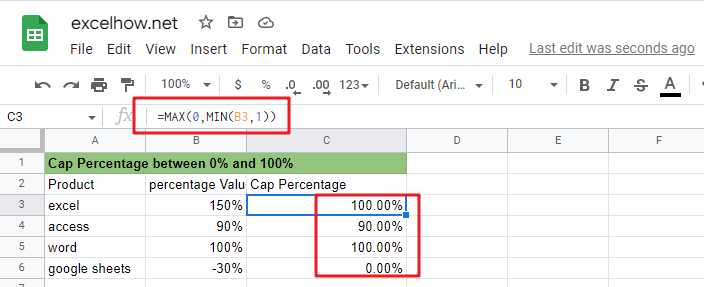If you are a newbie on Excel or google Sheets, you may be able to do this by manually. For tasks with small amounts of data, this method is possible, but for tasks with large amounts of data, this is not a wise method because it will take a lot of your time.

How to quickly cap a set of percentage values from 0% to 100%? You can now read the following section and we will talk about how to do this with formulas from Microsoft Excel or google sheets.

## Limiting percentages by MAX and MIN functions

You can use the MIN function in Google Sheets or Microsoft Excel Spreadsheet in combination with the MAX function to cap a given percentage value between 0% and 100%. Or you can do it with the IF nested functions.

Suppose we have a percentage value of 50%, which is greater than 0 and less than 100%, so it should return that percentage value; if the given percentage value is 120%, it is greater than 100%, so it should return 100%; if the given percentage value is -50%, it is less than 0%, so it should return 0%.

The general formula to achieve this task is as follows.

`=MAX(0,MIN(B3,1))`By applying this formula, you can always cap the percentage value between 0% and 100%.

### Formula explanation

Let’s see how this formula works.

#### =MIN(B3,1)

MIN function can be used to cap a given percentage value greater than 100%, returning 100%.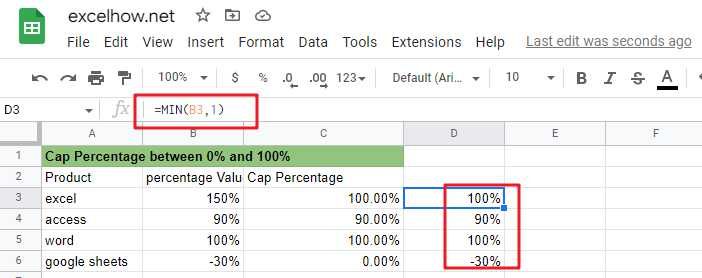#### =MAX(0,B3)

MAX function can be used to limit the given percentage value less than 0, return 0%.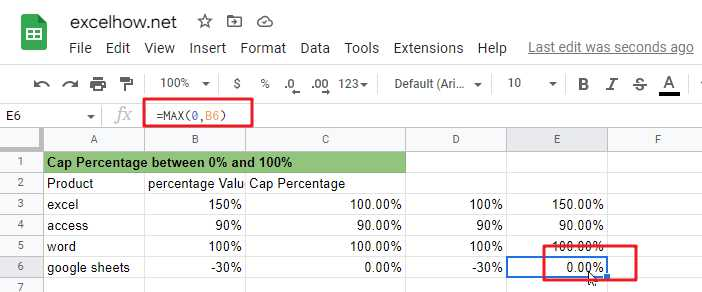## Limiting the percentage value by IF nested functions

You can also limit a given percentage value between 0% and 100% by using the IF nested function. The IF nested function is slightly more complex than the MAX/MIN nested function. There are three kinds of logic.

#### a) limit the value of the percentage does not exceed 100%

by the following IF formula can be used to limit the percentage value greater than 100%, so that the return value of 100%.

`=IF(B3>1,1,B3)`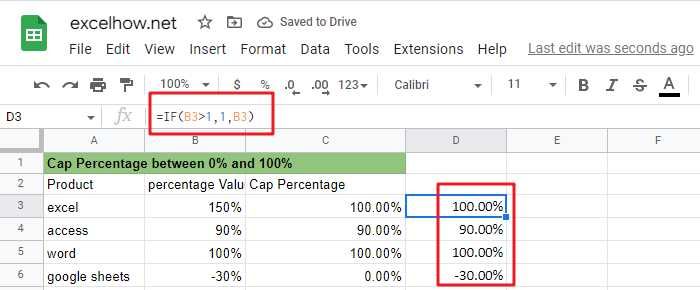#### b) Limit the percentage value not to be less than 0%

The following IF formula can be used to limit the percentage value less than 0, so that its return value is 0%

`=IF(B3<0,0,B3)`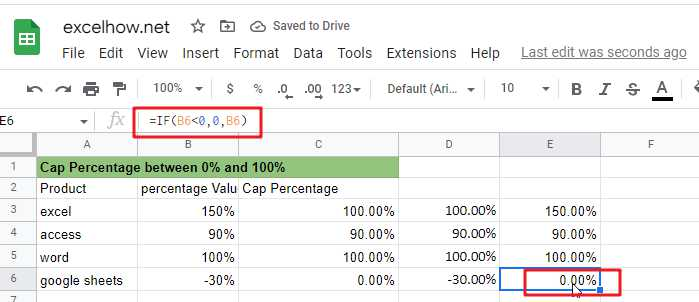#### c) Limit the percentage value that is greater than 0 and less than 100% and returns the original percentage value.

The above three selection logics can be satisfied simultaneously by the following nested IF formulas.

`=IF(B3>1,1,IF(B3<0,0,B3))`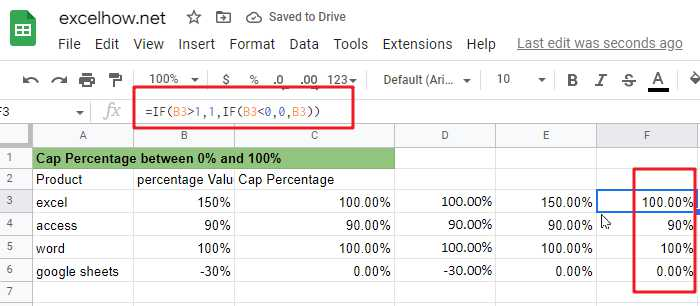From the screenshot above, you can see that the IF nested formula returns the same value as the MAX/MIN formula.

### Related Functions

The Google Sheets IF function perform a logical test to return one value if the condition is TRUE and return another value if the condition is FALSE. The IF function is a build-in function in Google Sheets and it is categorized as a Logical Function.The syntax of the IF function is as below:= IF (condition, [true_value],
The Google Sheets MIN function returns the smallest numeric value from the numbers that you provided. Or returns the smallest value in the array.The MIN function is a build-in function in Google Sheets and it is categorized as a Statistical Function.The syntax of the MIN function is as below:= MIN(num1,[num2,…numn])….
The Google Sheets MAX function returns the largest numeric value from the numbers that you provided. Or returns the largest value in the array.= MAX(num1,[num2,…numn])…

## Calculate Cap Percentages to Specific Value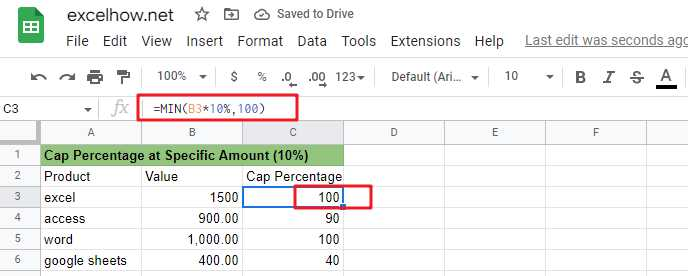If you are a newbie on Excel or google Sheets, you may be able to do this task manually. This method works for tasks with a small amount of data, but it is not a wise method for large amounts of data because it will consume a lot of your time.

How can you quickly calculate the cap percentage of a set of amount values? When you calculated the cap percentage of the amount, how do you limit that value to a given value? You can read the following section where we will explain how to do this with formulas in Microsoft Excel or google sheets.

## Calculate the cap percentage on a given amount

You can use the MIN function in Google Sheets or Microsoft Excel Spreadsheet to limit the cap percentage of a given amount to a specific value.

Suppose we have an amount value of 1500 and we need to calculate the upper limit of ten percent of that amount value and return 100 if the calculated cap value is greater than 100, or return that cap value if the calculated cap value is less than 100.

The general formula to complete this task is as follows.

`=MIN(B1*percentage,100)`

By executing this formula, you can always limit the cap percentage value of the amount value to 100.### Explanation of the formula

Let’s look at how this formula works：

Here are the three logics implemented in this formula.

• The MIN formula returns the cap percentage value when the calculated cap percentage value is less than 100.
• The MIN formula returns 100 when the calculated upper percentage value is greater than 100.
• The MIN formula returns 100 when the calculated upper percentage value is equal to 100.

From the above explanation, we can see that the MIN function can return the smallest value between the two values, but also can be used to achieve similar to the IF function can be used to select a specific value of the processing logic. Therefore, sometimes we can also use the MIN function instead of the IF function to complete our task.

### Related Functions

The Google Sheets IF function perform a logical test to return one value if the condition is TRUE and return another value if the condition is FALSE. The IF function is a build-in function in Google Sheets and it is categorized as a Logical Function.The syntax of the IF function is as below:= IF (condition, [true_value],
The Google Sheets MIN function returns the smallest numeric value from the numbers that you provided. Or returns the smallest value in the array.The MIN function is a build-in function in Google Sheets and it is categorized as a Statistical Function.The syntax of the MIN function is as below:= MIN(num1,[num2,…numn])….

## Find the Closest Data to the Data Provided in Google Sheets

In our daily work, we may encounter such an issue that to find the closest value to a certain value. In fact, google sheets internal functions can help us solve this problem. In today’s article, we will show you how to find the student whose score value is closet to the score provided with help of google sheets INDEX/MATCH/MIN/ABS functions.

Look at the following example, we want to know who has a score closest to score “150” or “100”.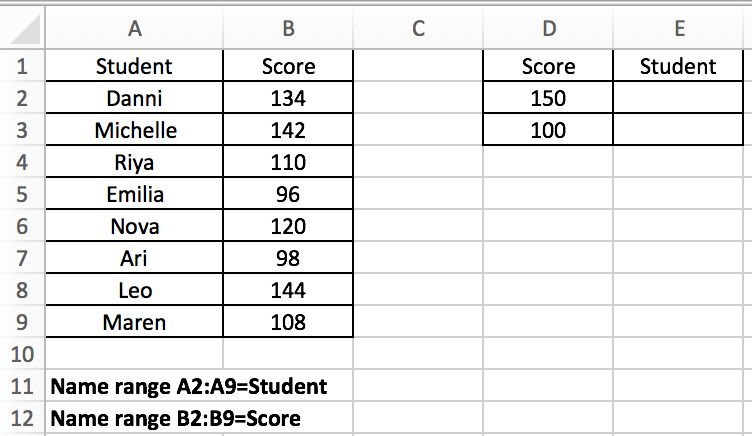Expect Result: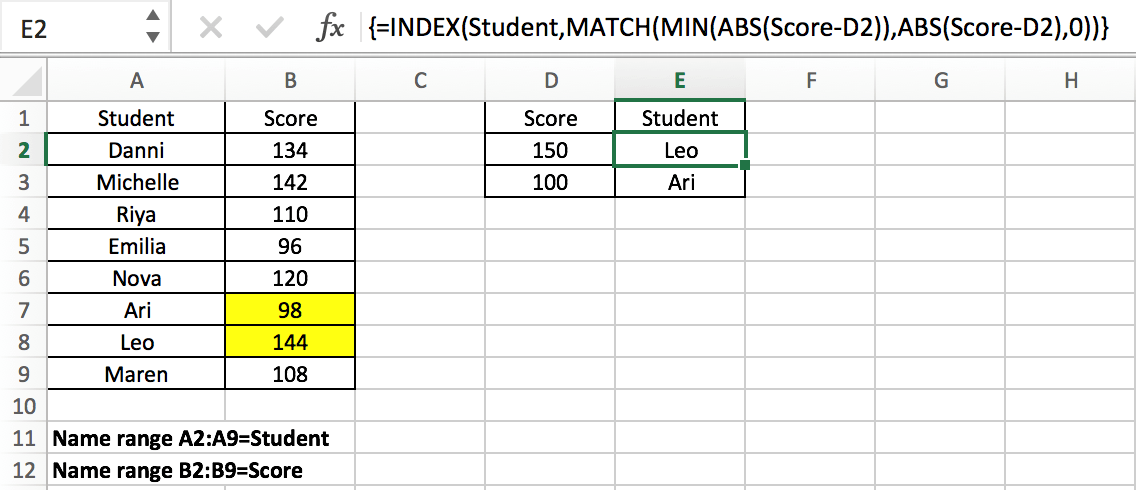## GENERAL FORMULA IN GOOGLE SHEETS

The general formula for this case is

`=INDEX(Data,MATCH(MIN(ABS(Data-ProvideValue)),ABS(Data-ProvideValue),0))`

In the general formula, you can replace Data and ProvideData with your own range or data reference. This is an array formula and we need to press “control + shift + enter” after entering the formula in google sheets .

In the above example, the formula is

`=INDEX(Student,MATCH(MIN(ABS(Score-D2)),ABS(Score-D2),0)).`

In the formula, Data is “Student” list (A2:A9, named “Student”); there are two provided values, 150 in D2 and 100 in D3; In this example, we want to find the closest score to the provided score (in D2 and D3) from the “Scores” list and retrieve the matching student name from the “Students” list that will be returned by the formula and filled in correctly in E2 and E3. Enter above formula in C8, then copy down the formula, the matching students are filled in properly.

Notes:

If we want to only obtain the score value instead of the student, we can just change the lookup array from Student to Score in this formula.

`=INDEX(Score,MATCH(MIN(ABS(Score-D2)),ABS(Score-D2),0))`

## EXPLANATION

For the formula, the core is the usage of google sheets  INDEX and MATCH functions combination. Before we can explain this formula, we need to know these two functions.

MATCH is an google sheets function for locating the position of a query value in a row, column, or table. INDEX is used to return the value at a certain position. As you can see, the MATCH function can provide a relative position of a value within a range, and INDEX can provide a suitable value based on the position provided, so typically, the MATCH and INDEX functions are used together to retrieve a value at a matching position.

### Syntax:

```=MATCH(lookup_value, lookup_array, [match_type]) (match type 0=exact match)

=INDEX(array, row_num, [column_num])```

In this example, using E2 as an example, we want to return the name of the student whose score retrieved from the student list is closest to the score 150 provided in D2, so for INDEX function in this formula, the array is the named range “Student” (A2:A9). We expand this array in the formula bar, the array is generated:

`{"Danni";"Michelle";"Riya";"Emilia";"Nova";"Ari";"Leo";"Maren"}`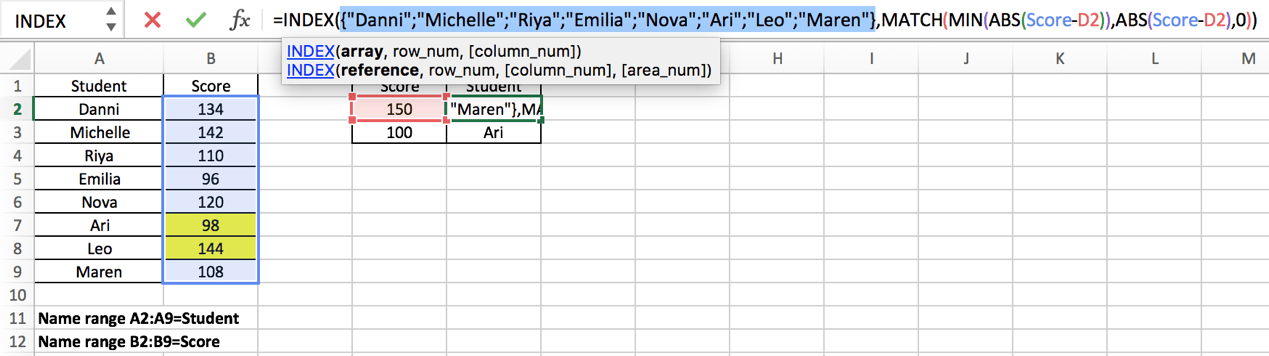In this INDEX function, the parameter “row_number” is obtained by executing another google sheets MATCH function. MATCH function will delivery its result to INDEX as row number. The hard work in this case is to find the closest score in Score list to the data provided in D column and obtain the relative position of this score in Score list. To resolve this issue, we use google sheets ABS and MIN functions in MATCH expression to obtain the minimum difference between the data and the data provided, and with their help, MATCH function can return the position of the closest score. To let you know how it works step by step, we will explain the expression from inside to outside as the result of the internal function will be delivered to the external one.

First, let’s get to know what the role ABS and MIN functions play in MATCH function.

ABS Function is used to return the absolute value of an integer.

Syntax:

`=ABS(value)`

MIN Function is used to return the smallest value in supplied data

Syntax:

`=MIN(number1, [number2], ...)`

In this example, MIN(ABS(Score-D2)) is the lookup value of the MATCH function. ABS(Score-D2) is the lookup array. Match type is 0, so the MATCH function returns an exact match.

Notes:

We all know that the smaller the difference between two values, the closer the two numbers are to each other. ABS(Score-D2) provides an array that save the differences between each value in “Score” and the value provided in D2, the differences may be negative or positive, but ABS function will convert the negative ones to positive numbers, so this function returns an array only contains positive numbers; And MIN(ABS(Score-D2)) provides the smallest difference among all differences.

For ABS(Score-D2), since the values saved in “Score” are vertically aligned, “Score” is a vertical array.; so (Score-D2) is also a vertical array. This step is done to get the difference between the two values.

Expand Score and D2 in the formula bar:

Score: {134;142;110;96;120;98;144;108}

D2:150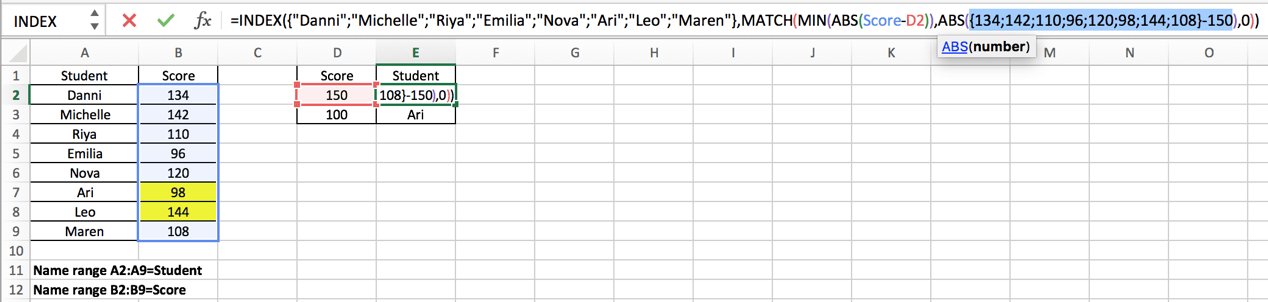Calculate (Score-D2) in google sheets formula bar and get below array:

`{-16;-8;-40;-54;-30;-52;-6;-42}`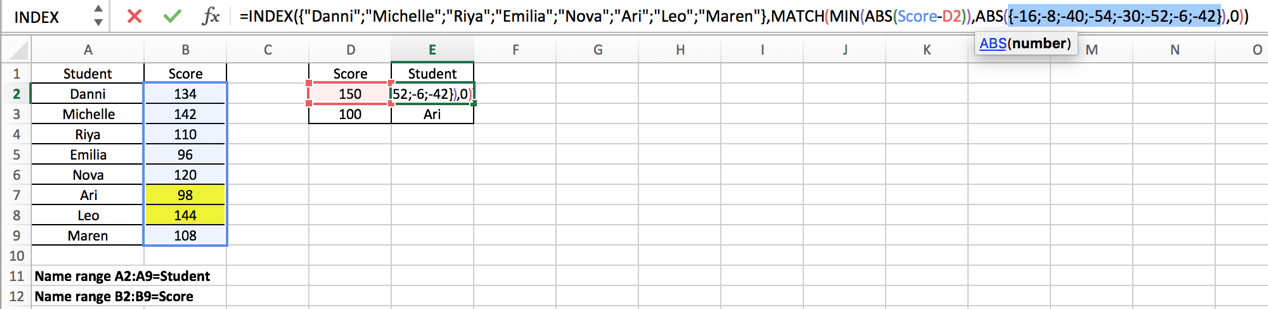Use google sheets ABS function to convert all negative numbers to positive numbers.

{16;8;40;54;30;52;6;42} – This array is the lookup array for MATCH function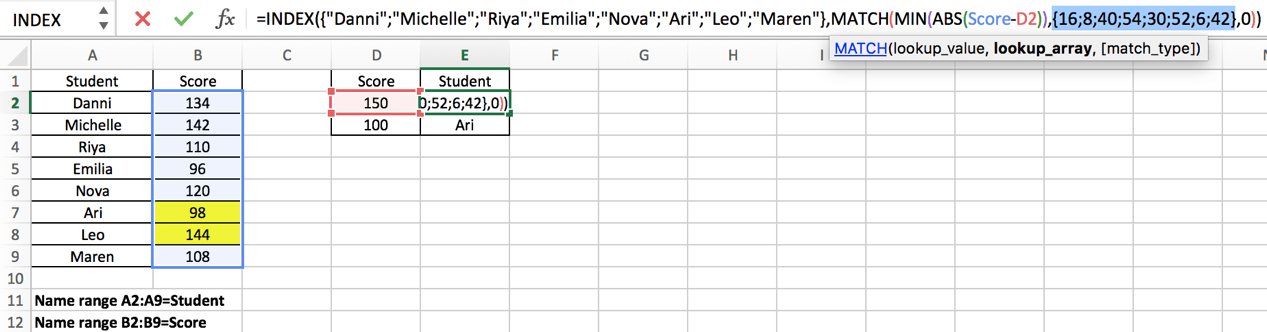For MIN(ABS(Score-D2)), the result of ABS function (numbers in the array above) is also delivered to MIN function:

### =MIN({16;8;40;54;30;52;6;42})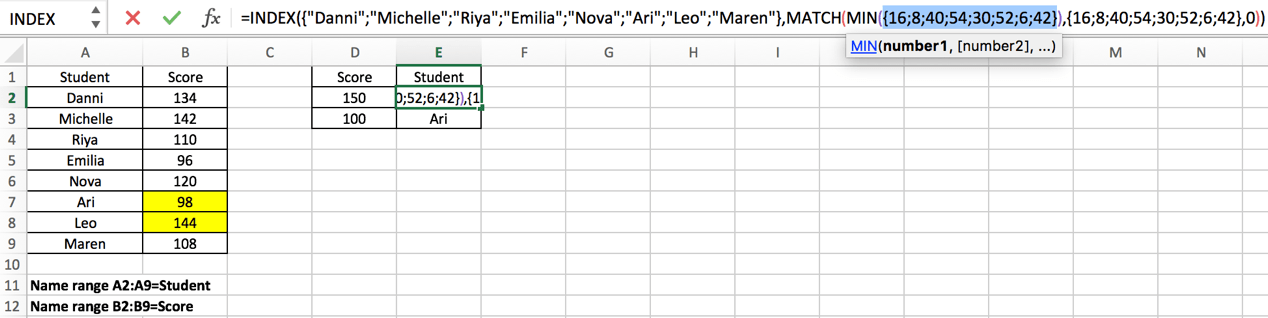The google sheets MIN function will extract the smallest value in the array.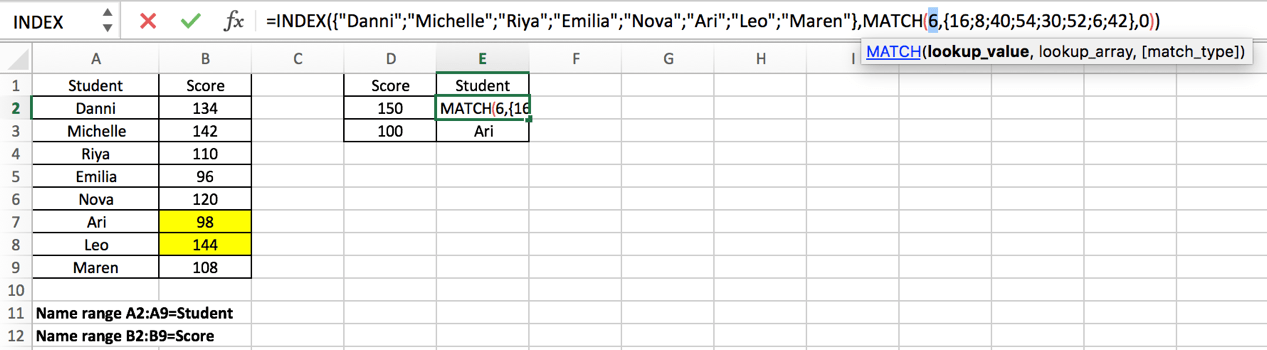Now, for MATCH function, the lookup value and lookup array are obtained after calculating ABS and MIN expressions.

### =MATCH(6,{16;8;40;54;30;52;6;42},0)

As mentioned above, google sheets MATCH function returns the position of a certain value, so in this case, MATCH function returns the row number of value “6” in array {16;8;40;54;30;52;6;42}. Obviously, relative to this array, 6 is in row 7.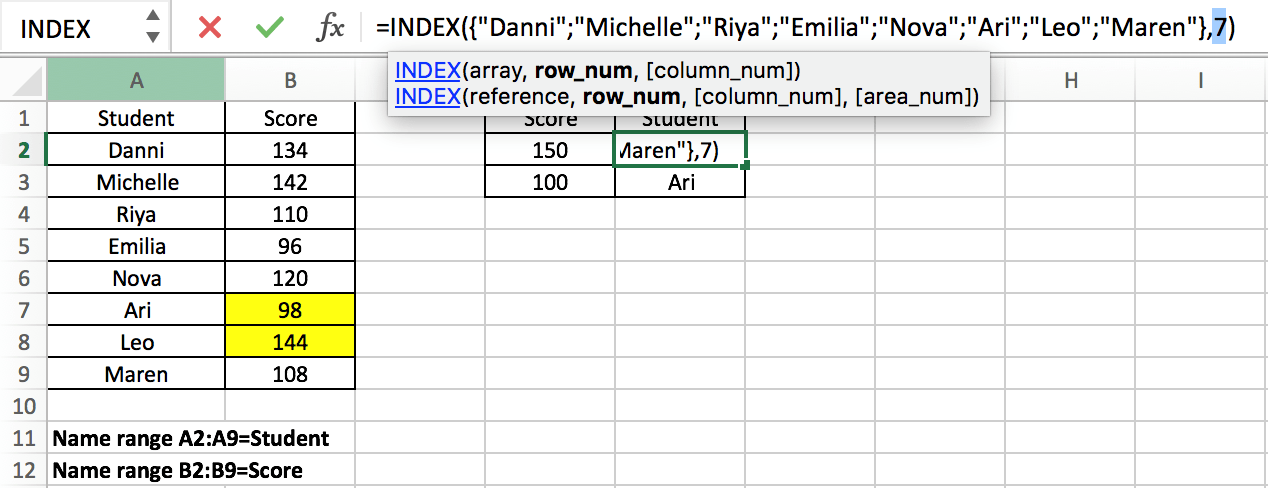Now we come to the outermost INDEX function：

`=INDEX({"Danni";"Michelle";"Riya";"Emilia";"Nova";"Ari";"Leo";"Maren"},7)`

In this formula, MATCH function delivered row number 7 to INDEX function, INDEX function returns a value in an array based on a provided row number. In this array which consists of student names, the seventh name is “Leo”, so this is the final result for this formula. After entering “Ctrl+Shift+Enter”, “Leo” is displayed in cell E2.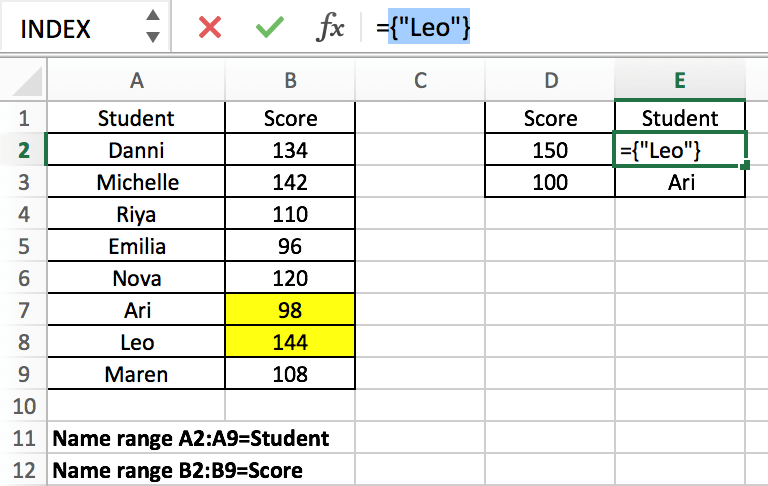Copied down the formula to E2, we can obtain “Ari” in the same way.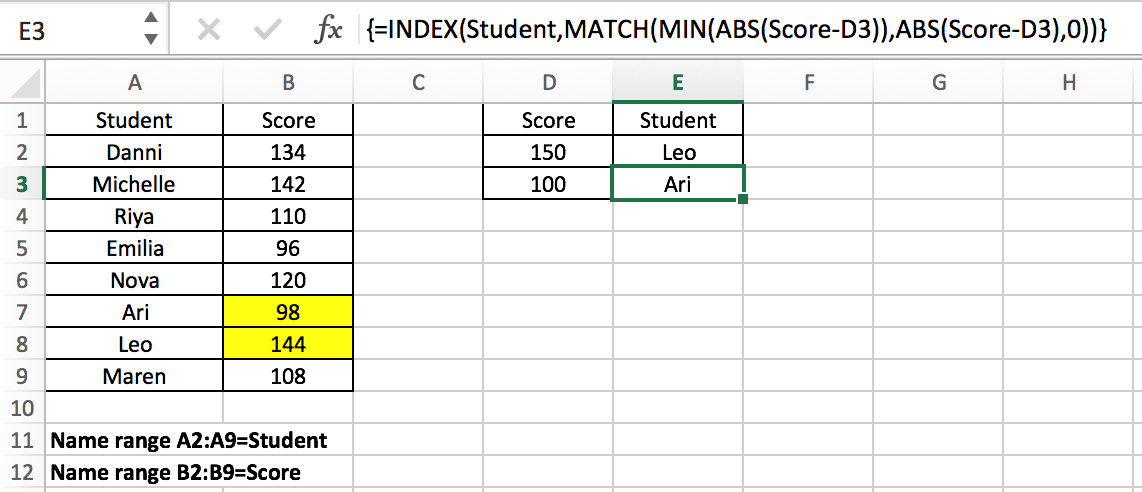This article not only introduces the joint application of INDEX and MATCH, but also the use of MIN and ABS functions, including how to apply the combination of MIN and ABS functions to find the minimum difference between values in google sheets . Readers can design their own formulas according to the actual situation.

### Related Functions

The Google Sheets INDEX function returns a value from a table based on the index (row number and column number)The INDEX function is a build-in function in Google Sheets and it is categorized as a Lookup and Reference Function.The syntax of the INDEX function is as below:= INDEX (array, row_num,[column_num])…
The Google Sheets MATCH function search a value in an array and returns the position of that item.The MATCH function is a build-in function in Google Sheets and it is categorized as a Lookup and Reference Function.The syntax of the MATCH function is as below:= MATCH  (lookup_value, lookup_array, [match_type])….
The Google Sheets MIN function returns the smallest numeric value from the numbers that you provided. Or returns the smallest value in the array.The MIN function is a build-in function in Google Sheets and it is categorized as a Statistical Function.The syntax of the MIN function is as below:= MIN(num1,[num2,…numn])….
The Google Sheets ABS function returns the absolute value of a number.The ABS function is a build-in function in google sheets and it is categorized as a Math and Trigonometry Function.The syntax of the ABS function is as below:=ABS (number)…

## Basic Array Formula With Examples in Google Sheets

Are you searching for an article for getting the basic array formula with different examples for better understanding? Then congratulations because you have just landed on the right article. In this article, you would get to know the basic array formula in google sheets and the use of this formula in different examples with a brief explanation.

#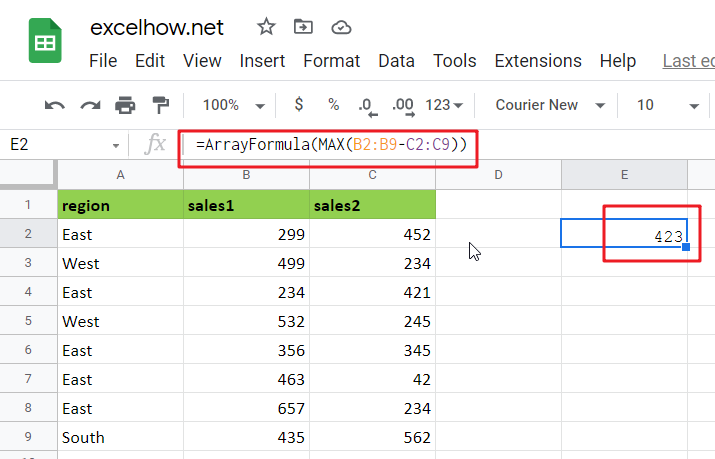## General Formula in Google Sheets

The basic array formula is given as follows:

{=MAX(range1-range2)}

### Syntax Explanation

• Minus Operator (-): This symbol is used to subtract any two values.
• Parenthesis (): The main function of this symbol is to group the elements.
• range1: This is the first input range in the Google sheet.
• Range2: This refers to the second input range.
• MAX: The MAX Function returns the largest numeric value from the range of input values.
• MIN: The MIN Function returns the smallest numeric value from a range of input values.

## Explanation

In Google Sheets, an Array Formula allows you to perform complex calculations on one or more value sets. The result could be a single cell or an array. An array is simply a list or range of values, but an Array Formula is a particular type of formula that must be entered by pressing Ctrl+Shift+Enter after that, the formula would be displayed in the formula bar surrounded by curly brackets {=…}

Array formulas are commonly used for data analysis, conditional sums and lookups, linear algebra, matrix math and manipulation, and many other tasks. A new Google Sheets user may come across array formulas in other people’s spreadsheets, but creating array formulas is typically an intermediate-to-advanced topic.

An array formula is commonly used to calculate the maximum or minimum change in a data set without using a helper column. In the example, the formula in E2 is mentioned as follows:

`=MAX(B2:B9-C2:C9)`

#This array formula must be entered with control + shift + enter.

This page’s example shows a simple array formula. Working from the inside out, the expression:

=B2:B9-C2:C9

As a result, an array of seven values is returned:

`{-153;265;-187;287;11;421;423;-127}`

#Each number in the array results from subtracting the “low” from the “high” in each of the seven rows of data. This array is returned to the MAX function:

=MAX({-153;265;-187;287;11;421;423;-127})

#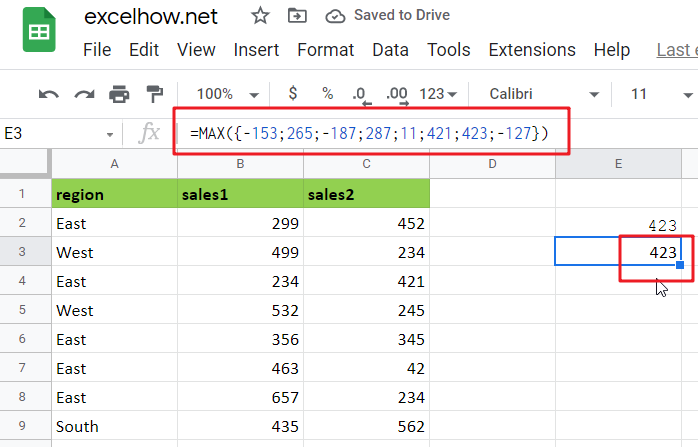And MAX returns the maximum value in the array, which is 423.

To return the smallest change in the data, substitute the MIN function for the MAX function:

`=MIN(B2:B9-C2:C9)`

#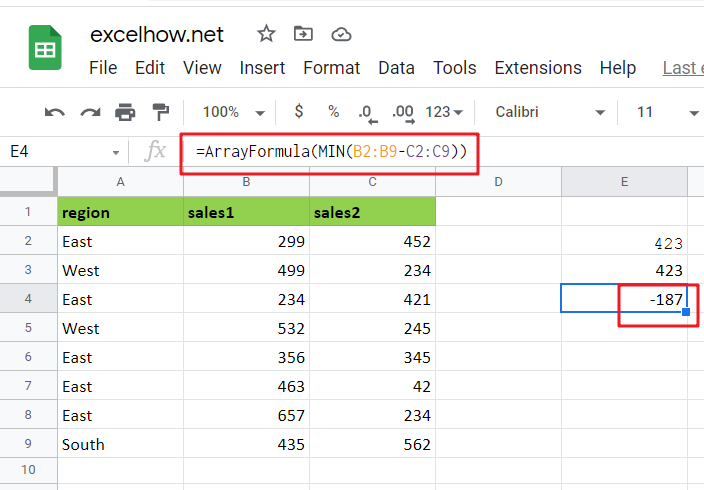As before, this is an array formula that must be entered with control + shift + enter.

## More Examples For Better Understanding in Google Sheets

For basic array formulas in Google Sheets, use the steps below.

In the following example, you must create a sample data set in Google Sheets.

Then, in the formula bar, use the following formula to count the maximum change in a data set.

=MAX(B2:B9-C2:C9)

After pressing Ctrl+Shift+Enter, you will see the MAX value in cell E2.

Then, in the formula bar, use the following formula to count the minimum change in a data set.

=MIN(B2:B9-C2:C9)

Finally, after pressing Ctrl+Shift+Enter, you will see the result for the MIN in cell F2.

## More On Array Formulas

To understand array formulas, you must first learn to investigate the results of various operations within a formula as Google Sheets evaluates it. You must understand how to use the F9 key to debug a formula and how to use Google Sheets’s Evaluate Feature.

## Summary

### Related Functions

The Google Sheets MIN function returns the smallest numeric value from the numbers that you provided. Or returns the smallest value in the array.The MIN function is a build-in function in Google Sheets and it is categorized as a Statistical Function.The syntax of the MIN function is as below:= MIN(num1,[num2,…numn])….
The Google Sheets MAX function returns the largest numeric value from the numbers that you provided. Or returns the largest value in the array.= MAX(num1,[num2,…numn])…

## Calculate Average Of Last 5 Or N Values In Columns in Google Sheets

Suppose you come across a task where you need to calculate the average of the last 2 or 3 numeric values in google sheets, then what would you do?

If you are new to google sheets, then your first attempt might be doing this task manually, which is an acceptable way but only when the values of which you want to calculate the average is limited to 2 or 3 but when it comes to calculating the last 5 or N values in the columns then it becomes nearly impossible to do this cumbersome task on time!

Don’t worry about it because after reading this article, you will know the easiest way to calculate the average of the last 5 or N values from the columns within seconds.

So, let’s dive into the article.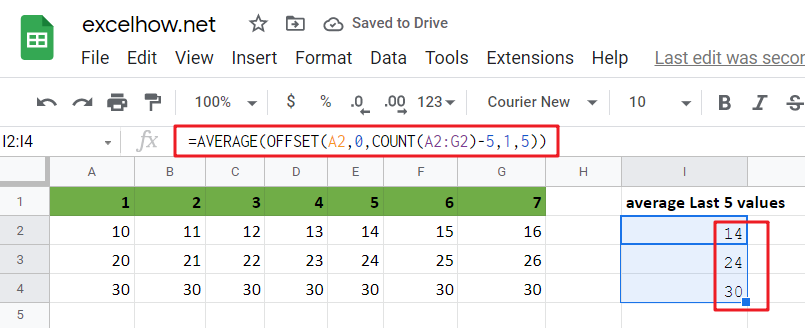## General Formula in google sheets

To get the average of the last N values in google sheets, use the formula below.

`=AVERAGE(OFFSET(first cell,0,COUNT(range_values)-N,1,N))`

### Explanations For Syntax:

• AVERAGE: In google sheets, the AVERAGE Function may be used to calculate the arithmetic mean of a set of integers.
• OFFSET: This Function outputs a reference to a range made up of pieces such as a beginning point, a row, and column offset, and a final height and width in rows and columns. Learn more about the OFFSET Function.
• COUNT: The COUNT Function returns the result as a number and counts the number of cells that contain numbers.
• First Cell: It indicates the first cell in the provided input range.
• The comma sign (,): is a separator that separates a list of values.
• Parenthesis (): The main Function of this symbol is to group the elements.
• Minus Operator (-): This symbol subtracts 2 values.

### Explanation

To average the latest 5 data values in a range of columns in google sheets, use the AVERAGE Function conjunction with the COUNT and OFFSET functions. The formula in I2 in the example is as follows:

`=AVERAGE(OFFSET(A2,0,COUNT(A2:G2)-5,1,5))`The OFFSET function may create dynamic ranges from a beginning cell and specify rows, columns, height, and width.

The rows and columns parameters act as “offsets” from the beginning reference. The height and width parameters, all optional, decide how many rows and columns are included in the final range. We want the OFFSET function to return a range that starts at the last item and grows “backward,” therefore we provide the following arguments:

A2 is the initial reference — it is the cell directly to the right of the formula and the first cell in the range of values we are dealing with.

Rows – We use 0 for the rows option because we want to stay in the same row.

Columns – We use the COUNT function to count all values in the range for the columns input, then deduct 5. This moves the start of the range of 5 columns to the left.

Height – we use 1 since we want a 1-row range as the end result.

Width – we pick 5 since we want a final range with 5 columns.

For the formula in I2, OFFSET yields a final range of C2:G2. This is sent to the AVERAGE Function, which returns the average of the five values in the range.

To understand better, consider the following example, which follows a step-by-step procedure:

• Consider the following example to calculate the average of the last 5 values.
• This illustration will provide input data from Column A to Column G.
• Next, enter the supplied formula in the formula bar section.
• Finally, we shall obtain the result in the selected cell I2.

## Less Than 5 Values Average in google sheets

If you are want to compute the average of the last 2, 3, or 4 data in google sheets, you don’t need to do it manually. Utilizing the formula discussed above will result in a circular reference mistake when computing the average of the 2, 3, or 4 values in the columns; the range will extend back into the cell that contains the formula. To avoid this mistake and complete your task, modify the formula as follows:

`=AVERAGE(OFFSET(first,0,COUNT(rng)-MIN(N,COUNT(rng)),1,MIN(N,COUNT(rng))))`

So If you want to average last N values in columns that less than 5 values in google sheets, you can use the following formula:

`=AVERAGE(OFFSET(A2,0,COUNT(A2:C2)-MIN(5,COUNT(A2:C2)),1,MIN(5,COUNT(A2:C2))))`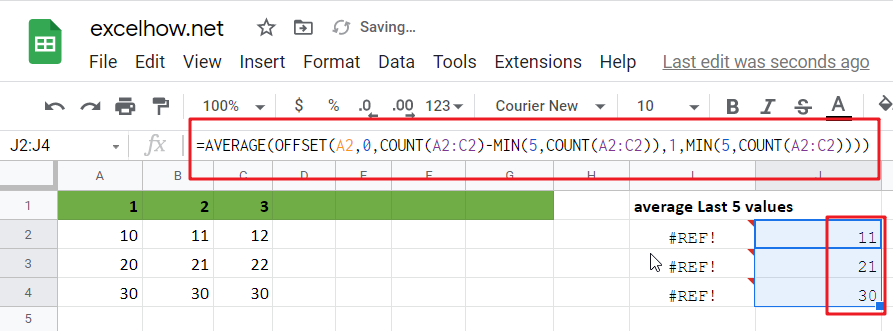In this case, we utilize the MIN function to “catch” cases where there are fewer than 5 values and the real count when there are.

### Related Functions

The Google Sheets AVERAGE function returns the average of the numbers that you provided.The syntax of the AVERAGE function is as below:=AVERAGE (number1,[number2],…)….
The Google Sheets  COUNT function counts the number of cells that contain numbers, and counts numbers within the list of arguments. It returns a numeric value that indicate the number of cells that contain numbers in a range…
The Google Sheets OFFSET function returns a range reference shifted a specified number of rows and columns from a starting cell reference.The syntax of the OFFSET function is as below:= OFFSET(cell_reference, offset_rows, offset_columns, [height], [width])…
The Google Sheets MIN function returns the smallest numeric value from the numbers that you provided. Or returns the smallest value in the array.The MIN function is a build-in function in Google Sheets and it is categorized as a Statistical Function.The syntax of the MIN function is as below:= MIN(num1,[num2,…numn])….

## Calculate The Average Of The Last 3, 5, Or N Numeric Values In Google Sheets

As an Google sheets user, you might have come across a task in which you need to calculate the average values of the last 2 numeric values, and you might have done this task manually but suppose if the last values you want to calculate the average exceeds to 3, 5 or N numeric values then what would you do? If you would tend to do this task manually, then it would be your foolish decision because doing these kinds of lengthy tasks manually is very hard, and when it comes to N numeric values, then It would not be wrong to say that this is near to impossible to do it manually.

But don’t worry about it because after carefully reading this article, you can easily calculate the average values of the last 3, 5, and most important N numeric values within seconds.

So without any further delay, let’s dive into it;

## Calculate The Average Of The Last 3 Values In Google Sheets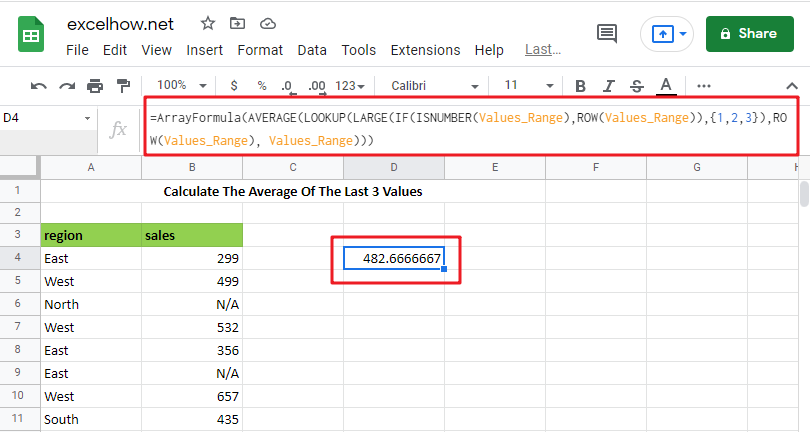The General Formula is as below based on the AVERAGE function, LOOKUP function, LARGE function and IF function:

`=AVERAGE(LOOKUP(LARGE(IF(ISNUMBER(Values_Range),ROW(Values_Range)),{1,2,3}),ROW(Values_Range), Values_Range))`

Note: this above is an array formula, you need to press “CTRL+SHIFT+ENTER” short cuts to make it as array formula.

## Let’s See How This Formula Works

You can use an array formula for getting the average of the last 3 values in the range. The formula in E3 in the example is as follows:

`=AVERAGE(LOOKUP(LARGE(IF(ISNUMBER(Values_Range),ROW(Values_Range)),{1,2,3}), ROW(Values_Range), Values_Range))`Here “ Values_Range ” refers to the designated name range B4:B11.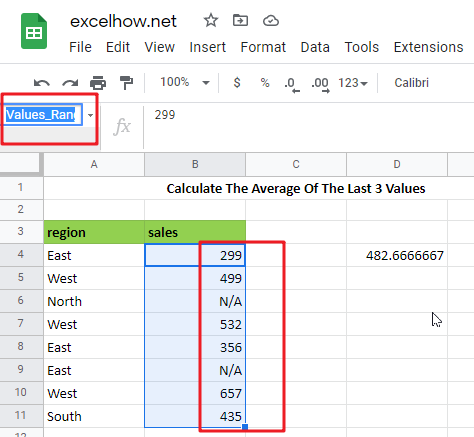This is an array formula, so input it using control + Shift + enter.

Because the AVERAGE function computes an average of numbers supplied in an array, practically all of the effort in this formula is to construct an array of the latest three numeric values in a range.

=IF(ISNUMBER(Values_Range),ROW(Values_Range))

The IF function in the formula is used to “filter” numeric numbers from the inside out.

Because the ISNUMBER function returns TRUE for numeric values and FALSE for other values (including blanks), and the ROW function produces row numbers, the outcome of this operation is an array of row numbers corresponding to numeric entries:

`={4;5;FALSE;7;8;FALSE;10;11}`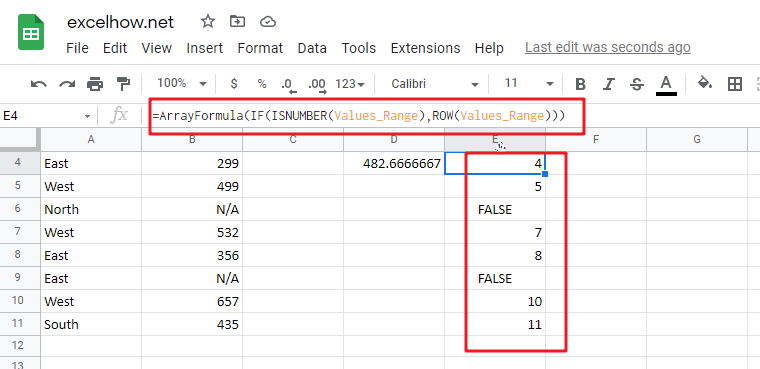=LARGE({4;5;FALSE;7;8;FALSE;10;11},{1,2,3})

The above array is sent to the LARGE function, using the array constants 1,2,3 for k. LARGE ignores FALSE values and returns an array with the greatest three integers, which correspond to the last three rows with numeric values:

`={11,10,8}`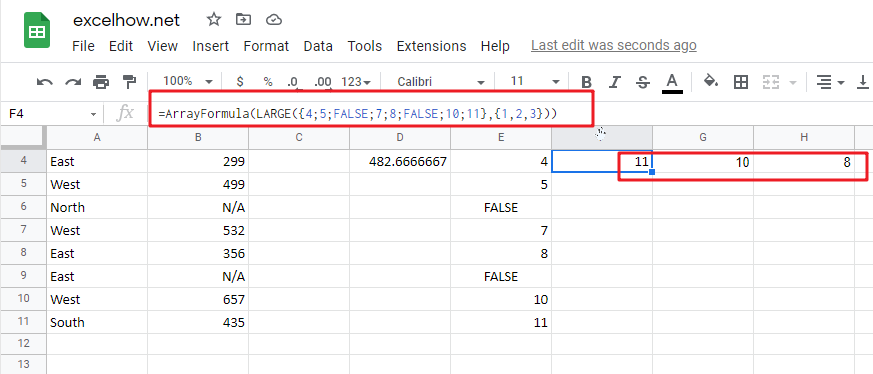=LOOKUP({11,10,8}, ROW(Values_Range), Values_Range))

The above array is used as the lookup value in the LOOKUP function. The ROW function provides the lookup array, and the return array is the named range “ Values_Range “:

`={435,657,356}`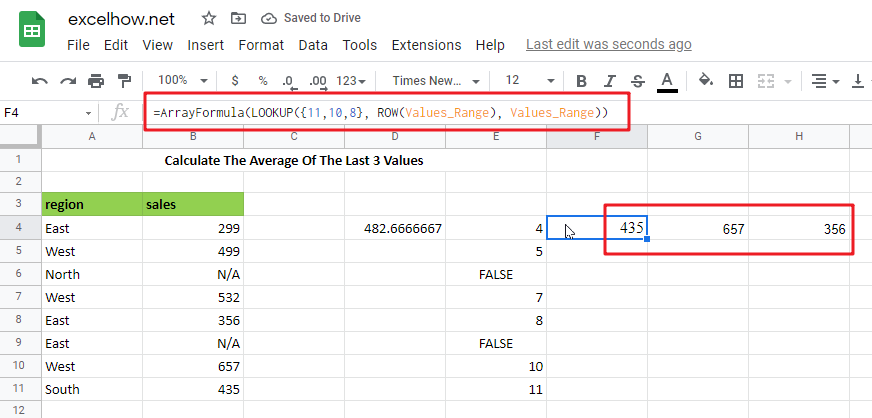The array of similar values in “ Values_Range ” returned by LOOKUP is then put into AVERAGE:

`=AVERAGE({435,657,356})`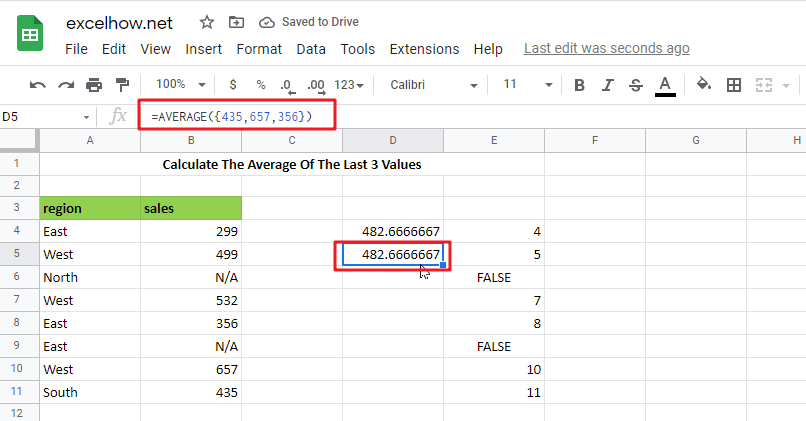## Calculate The Average Of The Last 5 Values IN Google Sheets

Please use the following array formula, which would assist you in calculating the last 5 values in Google Sheets:

In a blank cell, type the following formula:

`=IF(COUNT(B:B),AVERAGE(INDEX(B:B,LARGE(IF(ISNUMBER(B1: B10000),ROW(B1: B10000)),MIN(5,COUNT(B1: B10000)))):B10000),"no data found")`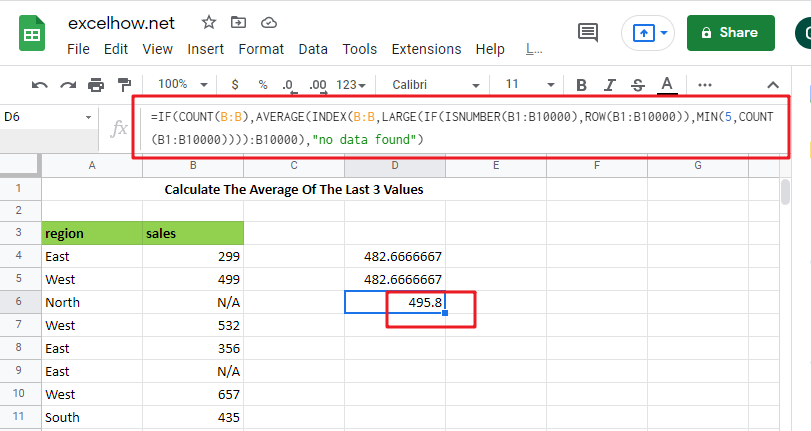Note: B:B is the column that holds the data you used, B1: B10000 is a dynamic range that you may stretch as long as you need, and the number 5 represents the latest n values, and then press Ctrl + Shift + Enter to make the formula as array formula to obtain the average of the last 5 numbers. See the following screenshot:

And now, when you enter new numbers underneath the old data, the average is updated as well, as seen in the screenshot: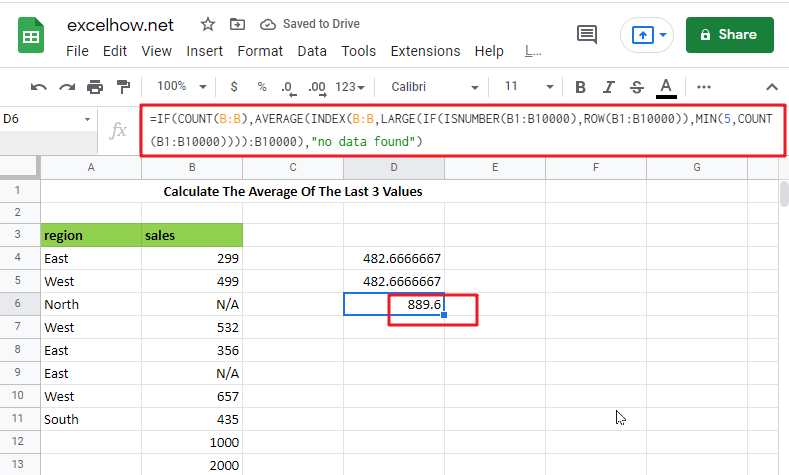### Related Functions

The Google Sheets ROW function returns the row number of a cell reference.The ROW function is a build-in function in Google Sheets and it is categorized as a Lookup and Reference Function.The syntax of the ROW function is as below:= ROW ([reference])….
The Google Sheets IF function perform a logical test to return one value if the condition is TRUE and return another value if the condition is FALSE. The IF function is a build-in function in Google Sheets and it is categorized as a Logical Function.The syntax of the IF function is as below:= IF (condition, [true_value],
The Google Sheets ISNUMBER function returns TRUE if the value in a cell is a numeric value, otherwise it will return FALSE.The syntax of the ISNUMBER function is as below:= ISNUMBER (value)…
The Google Sheets LOOKUP function will search a value in a vector or array.The syntax of the LOOKUP function is as below:= LOOKUP (lookup_value, lookup_vector, [result_vector])…
The Google Sheets AVERAGE function returns the average of the numbers that you provided.The syntax of the AVERAGE function is as below:=AVERAGE (number1,[number2],…)….
The Google Sheets LARGE function returns the largest numeric value from the numbers that you provided. Or returns the largest value in the array. The syntax of the LARGE function is as below:= LARGE (array,nth)…
The Google Sheets COUNT function counts the number of cells that contain numbers, and counts numbers within the list of arguments. It returns a numeric value that indicate the number of cells that contain numbers in a range…
The Google Sheets INDEX function returns a value from a table based on the index (row number and column number)The INDEX function is a build-in function in Google Sheets and it is categorized as a Lookup and Reference Function.The syntax of the INDEX function is as below:= INDEX (array, row_num,[column_num])…
The Google Sheets MIN function returns the smallest numeric value from the numbers that you provided. Or returns the smallest value in the array.The MIN function is a build-in function in Google Sheets and it is categorized as a Statistical Function.The syntax of the MIN function is as below:= MIN(num1,[num2,…numn])….
The Google Sheets ROW function returns the row number of a cell reference.The ROW function is a build-in function in Google Sheets and it is categorized as a Lookup and Reference Function.The syntax of the ROW function is as below:= ROW ([reference])….
The Google Sheets SUBTOTAL function returns the subtotal of the numbers in a list or database. The syntax of the SUBTOTAL function is as below:= SUBTOTAL (function_num, ref1, [ref2])….

This post will guide you how to use Google Sheets MIN function with syntax and examples in Google Spreadsheet.

## Description

The Google Sheets MIN function returns the smallest numeric value from the numbers that you provided. Or returns the smallest value in the array.

The MIN function is a build-in function in Google Sheets and it is categorized as a Statistical Function.

## Syntax

The syntax of the MIN function is as below:

= MIN(num1,[num2,…numn])

Where the MIN function arguments are:

• num1 -This is a required argument. A number, or a reference to a number or a range that contains numeric values
• Num2,…Numn – This is an optional argument.  A number, or a reference to a number or a range that contains numeric values

Note:

• The MIN function will not compare empty cells
• The MIN function can accept up to 255 arguments with numeric value.
• The MIN function will ignore Boolean values (TRUE and FALSE)
• The MIN function will ignore numbers that the format is text.
• The returned value of The MIN function is a numeric value.

## Google Sheets MIN Function Examples

The below examples will show you how to use Google Sheets MIN Function to get the smallest value form the numbers.

#1 To get the smallest value from the column A, just using the following Google Sheets formula:

`=MIN(B:B)`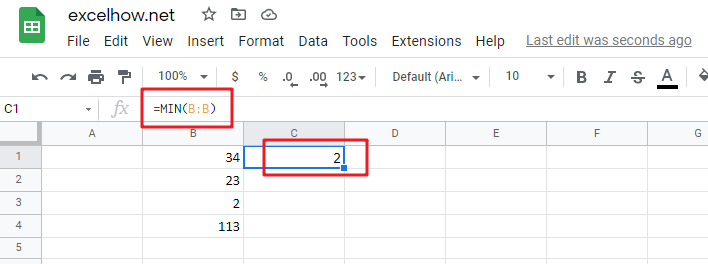Calculate The Average Of The Last 3, 5, Or N Numeric Values In Google Sheets

This post will guide you how to use Google Sheets IF function with syntax and examples in Google Spreadsheets.

## Description

The Google Sheets IF function perform a logical test to return one value if the condition is TRUE and return another value if the condition is FALSE.

The IF function is a build-in function in Google Spreadsheets and it is categorized as a Logical Function.

## Syntax

The syntax of the IF function is as below:

`= IF (condition, [true_value], [false_value])`

Where the IF function arguments are:

• `Condition` -This is a required argument.  A user-defined condition that is to be tested.
• `True_value` – This is an optional argument.  The value that is returned if condition evaluates to TRUE.
• `False_value` – This is an optional argument.  The value that is returned if condition evaluates to FALSE.

The below examples will show you how to use Google Sheets IF Function to return one value if the condition is TRUE or FALSE.

`=IF(B1>90, "good", "bad")`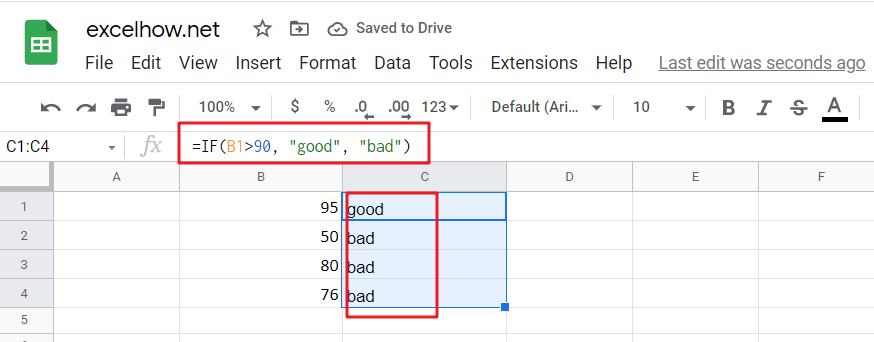Note: the above Google Sheets formula will test a condition “b1>90”, if the condition is true, then “good” will return or “bad” will return.

## Nested IF statements

The Google Sheets IF function just only test one condition and if you want to deal with more than one condition and return different actions depending on the result of the tests,  then you need to include several IF statements (functions) in one Google Sheets IF formula, these multiple IF statements are also called Google Sheets Nested IF formula(Nested IFs).

For example:

`=IF(A1>=80, "excellent", IF(A1>=60, "good", IF(A1>0, "bad", "no valid score")))`

When you are writing an If statement, you may need to use any of the following Google Sheets logical operators:

 Operator Meaning Example Description = Equal To A1=B1 Returns True if a value in cell A1 is equal to the values in cell B1; FALSE if they are not. > Greater Than A1>B1 Returns True if a value in cell A1 is greater than the values in cell B1; FALSE if they are not. >= Greater Than or Equal to A1>=B1 Returns True if a value in cell A1 is greater than or equal to the values in cell B1; FALSE if they are not. < Less Than A1 Not Equal to A1<>B1 Returns True if a value in cell A1 is not equal to the values in cell B1; FALSE if they are not.

Let’s see some examples for logical operators in Google Sheets formula:

 A1 B1 8 5
```  =(A1>B1)  //Output: TRUE

=(A1<B1) //Output: FALSE

=(A1>=B1) //Output: TRUE

=(A1<>8) //Output: FALSE

=(A1<>B1) //Output: TRUE

=(IF A1>3, “TRUE”, “FALSE”) //Output: TRUE```

Question1: I want to write an IF Formula based on the below test criteria in the Google Spreadsheets.

If the number in Cell B1 is greater than 30, then I want it to return A

If the number in cell B1 is between 20 and 30, then I want it to return B

If the number in Cell B1 is below 20, then I want it to return C

The below formula is what I currently write:

`=IF(B1>30,"A",IF(B1<30,B1>20,"B","C"))`

When I entered the above formula in the Cell C1, and it will return an Error.

Answer:  You can use IF function in combination with AND function to reflect the above logic. So you can do it using the following formula:

`=IF(B1>30,"A",IF(AND(B1>=20,B1<=30),"B","C"))`

Or you can use another IF formula without AND function as follows:

`=IF(B1>30,"A",IF(B1<20,"C","B"))`

Question 2: I want to write a Google Sheets Formula for sales rep, If the sales are less than 10K, then the member will get no commission. If the sales are between 10 and 50K, then the member will get a 3% commission. If the sales are more than 50k, then the member will get 5% commission. Could you help me?

Answer: Based on the above description, we can use the following Google Sheets IF formula:

`=IF(B1<10,0,IF(B1<50,B1*3%,B1*5%))`

Question 3: I want to select students for the scholarship in school, and based on the student’s scores and attendance value, if the student scores more than 85 and has the attendance of more than 85%, then he/she will get the scholarship. Please help me how to write this formula based on my test criteria.

Answer: you can use the IF function with the AND function to check whether both of two conditions are met or not. If the test are met, then return “Yes”, otherwise returns “No”.

So you can use the following IF formula in Google Sheets:

`=IF(AND(B1>85, C1>85%),"Yes","No")`

Question 4:  I am trying to write an Google Sheets formula based on the following logic:

I want to enter a formula in Cell C1 that will Add B1, B2 and B3 or multiply B1 by 1, multiply B2 by 2, multiply B2 by 3, which action will be taken based on what you put in Cell D1.

Appreciated for any help. Thanks

Answer: Based on the above logic description, you need to use SUM function to count the sum of B1, B2 and B3. We can use the following IF formula:

`=IF(D1="Add”,SUM(B1,B2,B3),IF(D1="Multiply",B1*B2*B3,"ERROR"))`

Question 5:  I am trying to write a formula in Google Sheets to check the employee number field and returns the relevant band of employee. I already have one IF formula, but I always return the first value of “A”. Could you help?

`=IF(B1>=10,"A",IF(B1>=20,"B",IF(B1>=50,"C")))`

Answer:  when you write an IF nested formula, you need to start with the largest number first or start the smallest number first with less than or equal to operator.

1# start with the largest number first, we can write down the below formula:

`=IF(B1>=50,"C",IF(B1>=20,"B",IF(B1>=10,"A")))`

2# start with the smallest number first, you need to change the >= to <= , just see the below formula:

`=IF(B1<=10,"A",IF(B1<=20,"B",IF(B1<=50,"C")))`

Question 6: I want to write a Formula in Google Sheets to return “bad” if the cell B1 is either <100 or >500, otherwise, it should be returned the value of cell B1.

Answer: For the above logic test, we should use the OR function in the IF condition, so we can write down the below IF formula:

`=IF(OR(B1>500,B1<100),"good",B1)`

Question 7: I want to write a formula in Google Sheets using IF function to check if the cell B1 >10 and B1<20. If TRUE, returns “good”, If FALSE, returns “bad”

Answer: You can use IF function in combination with AND function to check the value of Cell B1, if cell B1 is greater than 10 and cell B1 is less than 20, then returns “good”, otherwise, it will return “bad”.

`=IF(AND(B1>10,B1<20),"good","bad")`

Question 8:  I need to write a nested IF statement in Google Sheets to check the following logic:

If Cell B1 is less than 5, then multiply it by 5.

If Cell B1 is greater than or equal to 5 but less than 10, then multiply it by 10.

If cell B1 is greater than 10, then multiply it by 15.

Answer: This is a generic nested IF formula in Google Sheets, we can write the below nested IF statement to reflect the logic.

`=IF(B1<5, B1*5, IF(B1<10, B1*10, B1*15))`

Question 9:  I want to write a formula to match the following logic in Google Sheets:

If A1*B1 <=10, then returns A

If A1*B1 >10 but A1*B1 <=20, then returns B

If A1*B1 >20 but A1*B1 <=30, then returns C

If A1*B1>30, then returns D

Answer: You can use IF function to build a Nested IF statement in combination with AND function to achieve it. Let’s write down the following IF formula:

`=IF(A1*B1<=10,"A", IF(AND(A1*B1>10,A1*B1<=20),"B", IF(AND(A1*B1>20,a1*B1<=30,"C","D"))))`

Question 10: I want to write an IF function to check if the value in cell B1 is blank or Text string or Numeric value, if the cell B1 is empty, then return “blank”, if the cell B1 is a text string, then return “Text”, if the value in cell B1 is a numeric value, then return “number”. Any help for this formula, thanks.

Answer: Based on the description, you need to use ISBLANK function to check if the value in cell b1 is blank or not. And need to use ISTEXT function to check if the value in cell B1 is a text string or not and need to use ISNUMBER function to check if the value in cell B1 is a number or not. So you can write a nested IF statements in combination with ISBLANK, ISTEXT and ISNUMBER functions in Google Sheets as follows:

`=IF(ISBLANK(B1),"blank", IF(ISTEXT(B1),"Text", IF(ISNUMBER(B1),"number")))`

Question 11:  I want to write a formula in Google Sheets to calculate the bonus for employees in company, if the employee salary is greater than or equal to \$2000, then the bonus will be 10% of the salary , otherwise, the bonus will be 5% of the employee salary.

Answer:  we need to check if the salary in Cell B1 (it’s the salary of the first employee) is greater than or equal to 2000. It the condition test is TRUE, then returns B1*10%, otherwise returns b1*5%. So we can write down the following IF formula in Google Sheets:

`=IF(B1>=2000, B1*10%, B1*5%)`

Question 12: In Google Spreadsheets, I want to create an IF function to check if any employee who have at least 10 years of experience and whose salary is greater than \$5000, If TRUE, then the bonus will be 20% of salary.

Answer: you can use IF function in combination with AND function to check if the value in cell B1 is greater than or equal to 10 and the value in Cell C1 is greater than 5000. If both of two conditions are TRUE, then returns C1*20%, otherwise returns “No Bonus”.

Let’s write down the following IF statement as follows:

`=IF(AND(B1>=10,C1>5000),C1*20%, "No Bonus")`

Question 13: I want to create an IF formula in Google Sheets to check the following text logic:

If B1<10, then multiply B1 by 1%, but the returned value is not less than 50.

If B1>10, then multiply B1 by 2%, but the returned value is not greater than 100.

Answer: To reflect the first test condition, you need to use MAX function to match the condition. To reflect the second test condition, you need to use MIN function to match that the returned value should not be greater than 100. So we can use the following Google Sheets IF formula:

`=IF(B1<10, MAX(50,B1*1%), IF(B1>10, MIN(100,B1*5%)))`

Question 14:  I want to use IF function to check if B1 is greater than 10 and B2 is greater than 20 and B3 is less than 30, if TRUE, then returns “good”, otherwise, it should be returned “bad”.  So How to create the IF formula based on the above test criteria to check three conditions at the same time.

Answer: you need to use AND function within the IF function in Google Sheets to create an IF formula as follows:

`=IF(AND(B1>10,B2>20,B3<30),"good","bad")`

Question 15:  I have an IF formula that might cause a division by zero error, I don’t know how to avoid this kind of errors in Google Sheets formula.

Answer: You can use ISERROR function to catch this kind of errors then use the IF function to check the returned values from ISERROR function to avoid an error. So we can write an IF function in combination with ISERROR function in Google Sheets. For example, we can use the following IF formula:

`=IF(ISERROR(B1/C1),0, B1/C1)`

The ISERROR function will return TRUE when trying to divide B1 by 0.

Question 16:  I need to create a formula in Google Sheets to reflect the following logic:

If B1=sheets, then return W

If B1=info, then return A

Answer:  You can use IF function to create a nested IF statements as follows:

`=IF(B1=" Google ","G",IF(B1=" sheets ","S",IF(B1=" info ","I")))`

Question 17:  I have a worksheet that containing cells that are formatted as date format. I want to write an IF formula to check the first value in Cell (month part). So I am trying to create the following IF formula:

`=IF(LEFT(B1,1)=8, "August","Null")`

The returned results are always “Null”.

Answer: As the dates are not recognized as string, so you cannot use the LEFT function to exact the first value in the dates. At this moment, you need to use Month function to convert the date to its month number in Google Sheets. So we can write down the below IF formula in combination with Month function:

`=IF(MONTH(B1)=8, "August","Null")`

Question18: I am trying to create an IF formula to check if the time value in cells are greater than 10.00h for the following date format: 08/11/2018 09:43.50.  Appreciative of any help.

Answer: In Google Sheets, you can use HOUR, MINUTE, SECOND functions to compare the date or time value. so if you want to compare the value in Cell B1 if it is greater than 10h, then you can use HOUR function within IF function, just like this: HOUR(B1)>10, so we can write down the below formula:

`=IF(HOUR(B1)>10, "greater","less")`

Question 19: I want to create an IF function and need to combine with another RAND function.  The formula just works find for the first IF_VALUE_TRUE statement, but the formula works not good for IF_VALUE_FALSE statement. Here is the IF formula I have got:

`=IF(ISBLANK(B1),"","=rand()")`

In the above IF function, it will return empty string when the value in cell B1 is blank. Otherwise, it should add a random function, the problem is that the cell doesn’t run the RAND function. So how can I fix it?  Thanks

Answer:  when you write an IF formula, if you want to enclose others function, you need not to add quotes around the RAND () function, so just remove the quotes and equal sign in your IF function. Just like the below IF formula:

`=IF(ISBLANK(B1),"",RAND())`

Question 20: I am trying to write an IF formula in Google Sheets to reflect the following logic:

If B1 is greater than or equal to 50 and C1 is 0

OR

If B1 is greater than or equal to 30 and C1 is greater than or equal to 1

OR

If B1 is greater than or equal to 20 and C1 is 2

Then take the following action:  D2/E2

Otherwise, return FALSE

I have wrote the following IF formula, but it doesn’t work at all.

`=IF(AND(B1>=50,C1>=0),OR(AND(B1>=30,C1>=1)),OR(AND(B1>=20,C1>=2)),D2/E2,"FALSE")`

Answer: you need to nest your different AND function within an OR function in the IF formula. So you can try the below IF formula:

`=IF(OR(AND(B1>=50,C1>=0),AND(B1>=30,C1>=1),AND(B1>=20,C1>=2)), D2/E2,"FALSE")`

Question 21: I want to create an IF function in combination with MID function in Google Sheets. it need to check if the value in one specified cell is TRUE, then return the first six characters from another cell. Otherwise return empty value.

Answer: you just need to add MID function within IF function in Google Sheets, and do not add any quotes around MID function.    So you can use the following IF formula to achieve your request:

`=IF(B1=TRUE, MID(C1,1,5), "")`

Question 22:  I have a Google Sheets as below:

A     B

——

20   O

30   V

10   T

50   T

I want to create an IF formula to reflect the following logic:

IF cell A1 is less than or equal to 30 and Cell B1 is equal to “O” or “V”, if TRUE, then returns 300, otherwise returns 400.

IF Cell A1 is greater than 30 and Cell B1 is equal to “O” or “V”, then returns 500, otherwise returns 600.

I have wrote the below tow IF formulas for the above two conditions as follows:

```=IF(AND(A1<=30,OR(B1="O",B1="V")),300,400)

=IF(AND(A1>30,OR(B1="O",B1="V")),500,600)```

I am able to check the above two IF formula and the returned results is OK… But I am not able to combine the above two IF formula into a single IF formula. So anybody can help? Many thanks

Answer:  Based on the above logic, you can use the below IF formula to combine with above two IF formulas:

`=IF(A1<=30,IF(OR(B1="O",B1="V"),300,400),IF(OR(B1="O",B1="V"),500,600))`

Question 23: I am trying to write an IF function to prevent zero and negative values in cells. What I would like is that if the value in cell B1 is less than or equal to 0, then it should be returned “Null” otherwise, it should return the calculation of Cells value, like as:B2*(C2-D2)*E2.

The below IF formula is what I have:

`=IF(B1<=0,"Null","B2*(C2-D2)*E2")`

When I run the formula above, it only returns my calculation string and do not take the actual calculation.

Answer: In Google Sheets, the double quotes make any values in between be recognized as Text string. So if you want to take calculation for your IF formula, just remove the double quotes.  Let’s see the modified IF formula as follows:

`=IF(B1<=0,"Null",B2*(C2-D2)*E2)`

Question 24: I want to create an new IF function to check if the value in cells is Saturday or Sunday, If TRUE, returns “yes”, otherwise, returns “No”. And I am using the following IF formula, but I get an error, so what’s wrong for this formula?

`=IF((OR(\$B1="Saturday","\$B1="Sunday"),"yes","no"))`

Answer: you need to use the WEEKDAY function within IF function to handle the dates if they are in date format, like as: 11/8/2018.

So you can use the below IF function to achieve your logic:

`=IF(WEEKDAY(\$B\$1,2)>5,"yes","no")`

You can also use TEXT function within the IF function to achieve the same results, just like the below IF formula:

`=IF(LEFT(TEXT(\$B\$1,"ddd"))="S","yes","no")`

Question 25: I want to write an IF function in Google Sheets to check if the first character in one Cell is equal to 5, then the returned value should be the five rightmost characters of that cell, otherwise, the returned value should be the four rightmost characters. I wrote one IF formula as follows, but it doesn’t work.

`=IF(LEFT(B1,1)=5, RIGHT(B1,5),RIGHT(B1,4))`

In the above IF function, it always return the rightmost four characters even though the first character in Cell B1 starts with “S”.  Please help me to fix it.

Answer: you should know the returned value of the LEFT function firstly in Google Sheets. As the LEFT function will return a Text value, so you also need to provide a string for comparison, so adding quotes to enclose it. Just like the below IF function:

`=IF(LEFT(B1,1)="5", RIGHT(B1,5),RIGHT(B1,4))`

There is another way to achieve the same results, you can use NUMBERVALUE function to convert the result of LEFT function to a numeric value, like the below IF function:

`=IF(NUMBERVALUE(LEFT(B1,1))=5,RIGHT(B1,5),RIGHT(B1,4))`

Question 26:  I have 2 columns contain date and time or just only contain time. And I want to check if the times of column A is greater than the times of column B. the key issue may be that column A has the date and time.  I wrote the following IF formula to run it in Cell C1, but it returned the inaccurate results.

`=IF(A1>B1,"yes","no")`

Any help would be appreciated… Many thanks!

Answer:  the date part of the value in column A is the integer, while the time is the decimal. You can use the following IF formula:

`=IF(A1-INT(A1)>B2),"yes","no")`

Question 27:  The below are the results that I expected, and I want cell B1 to B4 can detect the string from A1 to A4 automatically and return the same string value plus the severity level when the test match. For example, If A1 is equal to “critical”, then it should be returned “critical severity 1” in the cell B1. Etc.

And I am using the following IF formula, but it does not work at all. Please help to fix it. Thanks

`=IF(A1="Critical","Critical Severity 1",""),IF(A1="High","High Severity 2",""),IF(A1="Medium","Medium Severity 3",""),IF(A1="Low","Low Severity 4","")`

 A B critical critical severity 1 high high severity 2 medium Medium severity 3 low low severity 4

Answer: you can try to run the following IF formula in Google Sheets:

`=IF(A1="critical","critical Severity 1",IF(A1="high","high Severity 2",IF(A1="medium","medium Severity 3",IF(A1="low","low Severity 4",""))))`

Question 28: I am working on a Google Sheets file and want to create a new IF formula to reflect the following logic:

If the value in Cell A1 is equal to the value in Cell A2, then check if the minus of B1 and B2 is equal to a special value, and if the condition is TRUE, returns “yes”, otherwise, returns “no”.  Here is the IF formula I have:

`=if(A1=A2,B1-B2=5 or B1-B2=-5 or B1-B2=20 or B1-B2=-20, "yes", "no")`

Any help is appreciated…Thanks

Answer: you need to use OR function with IF function to create a nested IF statement to achieve your request. So you can try the following Google Sheets IF formula in your Google Sheets file.

`=IF(A1=A2,IF(OR(B1-B2=5,B1-B2=-5,B1-B2=20,B1-B2=-20),"yes","no"),"no")`

Question 29: I want to create an IF formula to check the range of cells in Google Sheets.  I have scores of different subject for a student, and want to check if any one of scores is less than 60, If TRUE, then return “BAD”.  Can this logic be done with the IF statements in Google Sheets?

Answer: you can use COUNTIF function within IF function to create a generic IF formula as follows:

`=IF(COUNTIF(B:B,"<60")>0,"BAD","Good")`

Question 30: I am trying to create a new IF statement so that when the formula is looking at Row A and Row B, the returned values should be shown in the Row C. the following logic need to be checked:

IF the value in the Row A is equal to “NA” And the value in the Row B is equal to “NA”, then return “NA” value in Row C.

IF the value in the Row A is equal to “NA”, and the value in the Row B is equal to “denied”, then return “denied” value in Row C.

IF the value in the Row A is equal to “allowed” and the value in the Row B is equal to “NA”, then return “allowed” value in Row C.

Here is my formula:

`=IF(AND(A1 = "NA", B1 = "NA"),"NA",IF(OR(A1="denied",B1 ="denied"),"denied", "NA"))`

I don’t know how to include “allowed” in the IF formula above, anyone can help this, many thanks.

Answer: This is a typical nested if statement, you can use OR function within IF function in Google Sheets. We can write down this nested IF formula as follows:

`=IF(OR(A1="denied", B1="denied"), "denied", IF(OR(A1="allowed", B1="allowed"), "allowed", "NA"))`